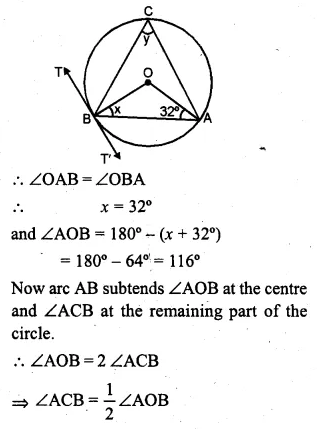Guru

# Question 39. (a) In the figure (i) given below, AB is a chord of the circle with centre O, BT is tangent to the circle. If ∠OAB = 32°, find the values of x and y

• 1

This is the basic and conceptual question from Chapter name- circles
Topic – Angle properties of circles
Chapter number- 15

In this question we have a figure in which it is given that , AB is a chord of the circle with centre O, BT is tangent to the circle.

Also ∠OAB = 32°,

Now using these facts we have to find the values of x and y

ICSE Avichal publication
Understanding ICSE Mathematics
Question 39(a)

Share